﻿ 分形树状微通道散热性能的数值研究

# 分形树状微通道散热性能的数值研究Numerical Study on Heat Dissipation Performance of Fractal Tree Microchannel

Abstract: Biomimetic fractal tree microchannel has been widely studied because of its uniform velocity dis-tribution and high heat transfer efficiency. Under the condition of constant cross-sectional area and hydraulic diameter, four kinds of fractal tree microchannels were constructed according to the dif-ferent aspect ratios (0.333, 1, 2 and 4) of the entrance section of a single microchannel. The heat transfer, flow and comprehensive performance of the microchannel with different Re were numeri-cally studied. The results showed that the Nu and pressure drop ΔP of the microchannel increased with the increment of aspect ratio. However, the maximum temperature Tmax of the microchannel increased with the increase of Nu. Among them, DJM-0.333 and DSL-0.333 had the smallest Tmax and better heat dissipation performance. After analyzing the comprehensive performance, it was found that the COP of different microchannels all decreased with the increase of Re, DJM-0.333 had the highest COP, while DJM-4 (DSL-4) had the lowest COP, and the former is 37% higher than the latter on average. It was further concluded that DJM-0.333 had the best heat dissipation performance.

1. 引言

1981年，受自然界中网络结构与人体循环结构的启发，Bejan  首次提出了一种微通道网络结构，并将其称为分形微通道。截止目前，不少学者对分形树状微通道的传热和压降性能进行了大量的研究。徐国强等人  研究了Y形微通道的流动特性和散热特性，探究了Y形的分叉角度对于散热器性能的影响。发现Y形微通道散热器的换热效果优于直通道，且Y形散热器的压降更小。侯亚丽和汪建文  通过改变分形微通道结构参数，使用实验的方法研究了不同水力直径、深宽比和长径比对微通道内流体流动特性的影响。结果发现，深宽比的增加会增大摩擦系数，而水力直径和长径比的增加则会减小摩擦系数。Zhang等    对不同高宽比的分形微通道、S形微通道和长直微通道进行了研究，发现分形结构和弯曲结构都会增大压降，但也增强了换热效果，且更大的高宽比会导致更大的压降和更小的换热系数。Carlos  等人在前人研究基础上，进一步模拟研究了Ψ与Y形结构，发现与Ψ形结构相比，Y形结构具有更好的传热特性，且换热热阻更小，表面温度更均匀。谭慧等人  在植物叶脉结构的基础上，设计了一种叶脉型微通道热沉结构。与平行微通道比较，叶脉型传热效率更高，温度分布更为均匀，压力损失更小。Liang  等采用实验与数值相结合的方法研究了Y形分形微通道内的流动与传热，并提出了一种改进的新结构，进一步提高了微通道传热性能，并使底壁具有更均匀的温度分布。Huang  等人考虑到仿生分形与变截面的耦合效应，首次提出了具有可变截面的树形微通道(TMHS)，对其传热、流动以及综合性能进行了数值研究，并与传统的光滑TMHS进行了比较。结果表明，变截面结构可以进一步提高TMHS的传热性能，但同时也增加了压降。石瀚楠等  在给定脉管通道总体积和热沉上表面面积的约束条件下，对树状脉管通道热沉进行了数值研究，发现“辐射 + 树状”复合脉管通道的构形设计能进一步降低最大温差和当量热阻。

2. 数值模型

2.1. 物理模型

$\frac{{L}_{k+1}}{{L}_{\text{k}}}={n}^{-1/E}$ (1)

$\frac{{D}_{k+1}}{{D}_{k}}={n}^{-1/AC}$ (2)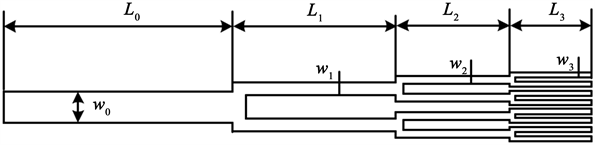Figure 1. Fractal tree-like single channel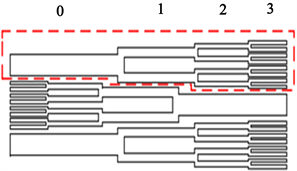(a)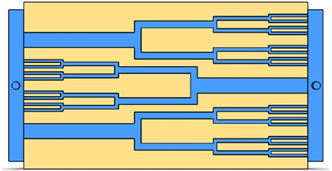(b)

Figure 2. Fractal treemicrochannel. (a) Schematic diagram of tree microchannel structure; (b) Physical modelTable 1. Specific structural parameters of each model under a fixed cross-sectional areaTable 2. Specific structural parameters of each model under constant hydraulic diameter

2.2. 控制方程

1) 流体是不可压缩的

2) 重力和粘性耗散的影响可以忽略不计

3) 可忽略的辐射热传递

4) 流体和固体材料特性是恒定的

$\frac{\partial \rho }{\partial t}+\frac{\partial \left(\rho {\mu }_{j}\right)}{\partial {x}_{j}}=0$ (3)

$\rho \frac{\partial k}{\partial t}+\rho \frac{\partial \left({\mu }_{j}k\right)}{\partial {x}_{j}}=\frac{\partial p}{\partial {x}_{j}}\left[\left(\mu +\frac{{\mu }_{i}}{{\sigma }_{k}}\right)\frac{\partial k}{\partial {x}_{j}}\right]+{\eta }_{t}\frac{\partial {\mu }_{i}}{\partial {x}_{j}}\left(\frac{\partial {\mu }_{i}}{\partial {x}_{j}}+\frac{\partial {\mu }_{j}}{\partial {x}_{i}}\right)-\rho \epsilon$ (4)

$\rho \frac{\partial \epsilon }{\partial t}+\rho \frac{\partial \left({\mu }_{j}\epsilon \right)}{\partial {x}_{j}}=\frac{\partial }{\partial {x}_{j}}\left[\left(\mu +\frac{{\mu }_{t}}{{\sigma }_{\epsilon }}\right)\frac{\partial \epsilon }{\partial {x}_{j}}\right]+{c}_{1}\rho S\epsilon -{c}_{2}\rho \frac{{\epsilon }^{2}}{k+\sqrt{v\epsilon }}$ (5)

${c}_{1}=\mathrm{max}\left[0.43,\frac{\eta }{\eta +5}\right];\text{\hspace{0.17em}}\text{\hspace{0.17em}}\eta =\frac{Sk}{\epsilon };\text{\hspace{0.17em}}\text{\hspace{0.17em}}S=\sqrt{2{S}_{i,j}{S}_{i,j}};\text{\hspace{0.17em}}\text{\hspace{0.17em}}{S}_{i,j}=\frac{1}{2}\left(\frac{\partial {\mu }_{i}}{\partial {x}_{j}}+\frac{\partial {\mu }_{j}}{\partial {x}_{i}}\right)$ (6)

${c}_{2}=1.9;\text{\hspace{0.17em}}\text{\hspace{0.17em}}{\mu }_{l}=\frac{{c}_{\mu }\rho {k}^{2}}{\epsilon };\text{\hspace{0.17em}}\text{\hspace{0.17em}}{\sigma }_{k}=1.0;\text{\hspace{0.17em}}\text{\hspace{0.17em}}{\sigma }_{\epsilon }=1.2$ (7)

$\rho \frac{\partial T}{\partial t}+\rho \frac{\partial \left({u}_{i}T\right)}{\partial {x}_{i}}=\frac{\lambda }{{c}_{p}}\frac{{\partial }^{2}T}{\partial {x}_{i}^{2}}$ (8)

2.3. 网格划分及边界条件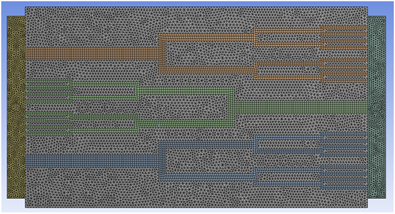Figure 3. Schematic diagram of fractal tree microchannel meshing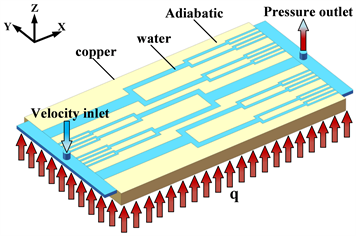Figure 4. Schematic diagram of boundary conditions

3. 数据处理

Re的计算公式表示：

$Re=\frac{{\rho }_{f}{u}_{in}{D}_{h}}{{u}_{f}}$ (9)

$Nu=\frac{{h}_{m}{D}_{h}}{{k}_{f}}$ (10)

${h}_{m}=\frac{{q}_{w}{A}_{q}}{{A}_{w}\left({T}_{w}-{T}_{m}\right)}$ (11)

${T}_{m}=\frac{{T}_{in}+{T}_{out}}{2}$ (12)

$COP=\frac{{Q}_{f}}{{P}_{p}}=\frac{{Q}_{f}}{\Delta p\cdot V}$ (13)

Qf表示流体吸收的热量， ${Q}_{f}=m{C}_{p}\left({T}_{out}-{T}_{in}\right)$ ，微通道进口质量流量 $m=V{\rho }_{f}=v{A}_{c}{\rho }_{f}$ ，v为流体进口速度，Ac表示微通道进口的横截面积，ρf为流体密度，Cp为流体的比热，Tout是流体出口的温度，Tin是流体进口的温度。ΔP为压降。V表示体积流率，V = vAc，v为流体进口速度，Ac表示微通道进口的横截面积。

4. 模型计算结果分析

4.1. 数值方法可靠性验证

4.1.1. 网格独立性验证Table 3. Grid independence verification results

4.1.2. 模型验证Figure 5. Comparison of simulation results with the experimental results of Qu et al.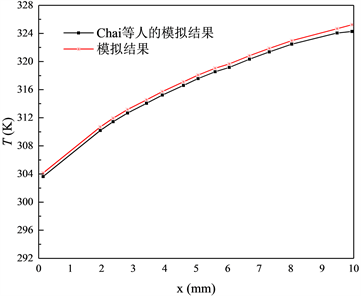Figure 6. Comparison of simulation results with those of Chai et al. 

4.2. 定横截面积下不同高宽比微通道的散热性能研究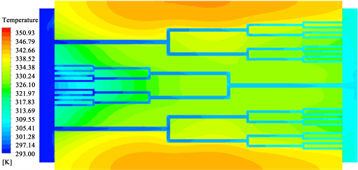(a) DJM-0.333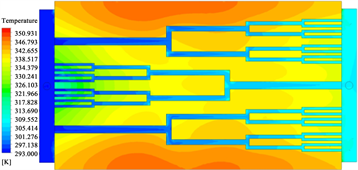(b) DJM-1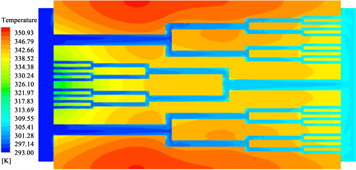(c) DJM-2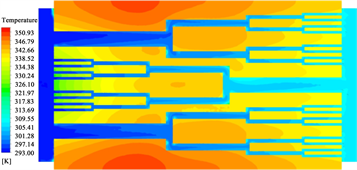(d) DJM-4

Figure 7. Temperature contours of the microchannel under a constant cross-sectional area

Nu随Re的变化如图9所示，随着Re的增大，Nu也随之增大。而在Re相等的情况下，散热器的Nu随着单条微通道进口截面高宽比的增加而增大，DJM-4的Nu最大，其入口流速达到18 m/s (Re = 17914)时，Nu达到最大值为42.44，DJM-0.333的Nu最小，为23.85。DJM-4的Nu在入口流速8 m/s (Re = 7962)增长到18 m/s (Re = 17914)的过程中，从23.06快速增加到42.44，DJM-4的Nu涨幅最大，而DJM-0.333的Nu在此过程中，从14.27缓慢增长到23.84，其中DJM-0.333的涨幅最小。

4种分形微通道的压降ΔP与Re的关系如图10所示，当Re增加时，ΔP也随之增加。Re ≤ 15,000时，ΔP增加的速率较小，Re > 15,000时，ΔP增速上升。在相同Re的条件下，微通道散热器的ΔP随着高宽比的增加而增大。其中DJM-0.333的ΔP最小，DJM-4的ΔP最大。入口速度为8 m/s (Re = 7962)时，DJM-0.333的ΔP为70.22 KPa，DJM-4的ΔP为96.16 KPa。在研究范围内，ΔP变化最小的是DJM-0.333，其最大ΔP为346.56 KPa，而DJM-4的ΔP变化最大，其最大ΔP为480.79 KPa。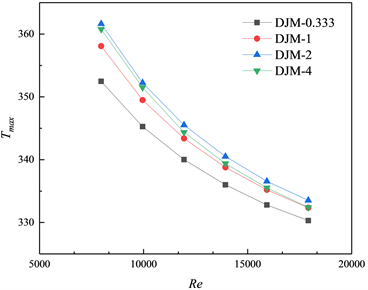Figure 8. The maximum temperature Tmax of the microchannel under different Re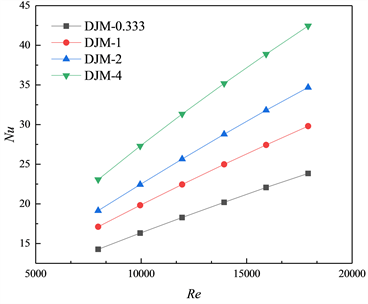Figure 9. Nu of microchannels under different Re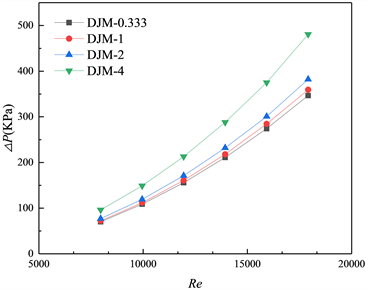Figure 10. ΔP of microchannels under different Re

4.3. 定水力直径下不同高宽比微通道的散热性能研究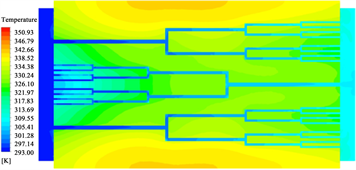(a) DSL-0.333(b) DSL-1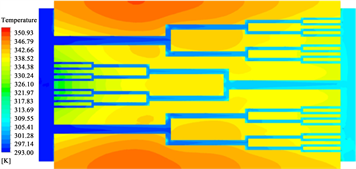(c) DSL-2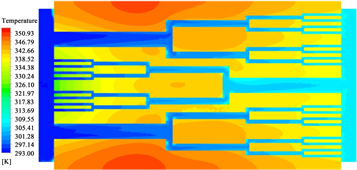(d) DSL-4

Figure 11. Temperature contours of microchannel under constant hydraulic diameter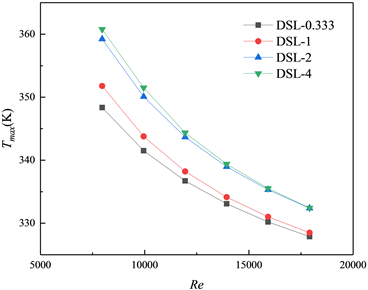Figure 12. The maximum temperature Tmax of the microchannel under different Re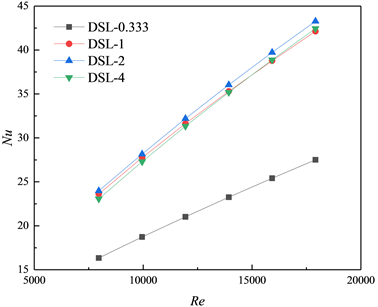Figure 13. Nu of microchannels under different Re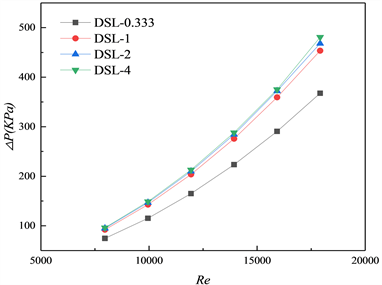Figure 14. ΔP of microchannels under different Re

4.4. 综合性能研究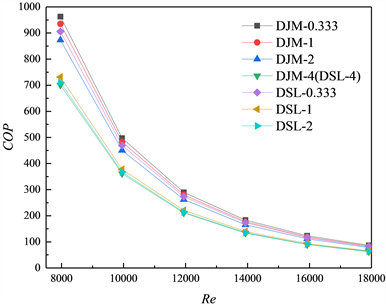Figure 15. Coefficient of performance COP of microchannel under different Re

5. 结论

1) 在单条通道定截面与定水力直径的条件下，通过改变高宽比，设计了7种不同分形树状微通道结构，对比分析了其传热和流动特性。结果表明，定截面与定水力直径下，微通道的Nu和压降ΔP均随着高宽比的增加而增大。然而由于总传热面积随高宽比的增加而减小，微通道的最高温度Tmax并没有随Nu的增加而降低，反而随Nu的增加而增加。相比于其他结构，DJM-0.333和DSL-0.333的Tmax更小，具有更好的散热性能。

2) 对得到的不同分形树状微通道的综合性能进行分析，发现不同微通道的COP均随Re的增加而减小，DJM-0.333具有最高的COP，而DJM-4 (DLS-4)的COP最小，且前者比后者平均高37%。因此，进一步得出DJM-0.333的分形树状微通道结构具有最佳的散热性能。

“高效节能的换热/反应装备关键技术”产学研合作项目(校企合作)。

NOTES

*通讯作者。

 Tuckerman, D.B. and Pease, R.F.W. (1981) High-Performance Heat Sinking for VLSI. Electron Device Letters, 2, 126-129.
https://doi.org/10.1109/EDL.1981.25367

 徐国强, 王梦, 吴宏, 等. Y形构形微通道流动换热特性的数值分析[J]. 北京航空航天大学学报, 2009, 35(3): 313-317.

 侯亚丽, 汪建文. 几何参数对矩形微通道内流体流动特性的影响[J]. 排灌机械工程学报, 2015(5): 417-421.

 Zhang, C.P., Lian, Y.F., Yu, X.F., et al. (2013) Numerical and Experimental Studies on Laminar Hydrodynamic and Thermal Characteristics in Fractal-Like Mi-crochannel Networks. Part A: Comparisons of Two Numerical Analysis Methods on Friction Factor and Nusselt Num-ber. International Journal of Heat and Mass Transfer, 66, 930-938.
https://doi.org/10.1016/j.ijheatmasstransfer.2013.06.073

 Zhang, C.P., Lian, Y.F., Yu, X.F., et al. (2013) Nu-merical and Experimental Studies on Laminar Hydrodynamic and Thermal Characteristics in Fractal-Like Microchannel Networks. Part B: Investigations on the Performances of Pressure Drop and Heat Transfer. International Journal of Heat and Mass Transfer, 66, 939-947.
https://doi.org/10.1016/j.ijheatmasstransfer.2013.07.021

 Zhang, C.P., Lian, Y.F., Hsu, C.H., et al. (2015) In-vestigations of Thermal and Flow Behavior of Bifurcations and Bends in Fractal-Like Microchannel Networks: Second-ary Flow and Recirculation Flow. International Journal of Heat and Mass Transfer, 85, 723-731.
https://doi.org/10.1016/j.ijheatmasstransfer.2015.01.118

 Rubio-Jimenez, C.A., Hernandez-Guerrero, A., Cer-vantes, J.G., et al. (2016) CFD Study of Constructal Microchannel Networks for Liquid-Cooling of Electronic Devices. Applied Thermal Engineering, 95, 374-381.
https://doi.org/10.1016/j.applthermaleng.2015.11.037

 谭慧, 宗宽, 熊长武, 等. 叶脉型微通道热沉设计及散热特性分析[J]. 工程设计学报, 2019, 26(4): 113-119.

 Liang, L.X., Hou, J.B., Fang, X.J., et al. (2019) Flow Characteristics and Heat Transfer Performance in a Y-Fractal Mini/Microchannel Heat Sink. Case Studies in Thermal Engineering, 15, Article ID: 100522.
https://doi.org/10.1016/j.csite.2019.100522

 Huang, P.N., Dong, G.P., Zhong, X.N., et al. (2020) Numerical Investigation of the Fluid Flow and Heat Transfer Characteristics of Tree-Shaped Microchannel Heat Sink with Variable Cross-Section. Chemical Engineering and Processing—Process Intensification, 147, Article ID: 107769.
https://doi.org/10.1016/j.cep.2019.107769

 石瀚楠, 谢志辉, 孙丰瑞, 等. 树状脉管圆盘热沉的传热分析与构形设计[J]. 工程热物理学报, 2019, 40(7): 140-145.

 Celata, G.P. and Carey, V.P. (2000) Heat Transfer and Transport Phenomena in Microscale. Proceedings of the International Conference on Heat Transfer and Transport Phenomena in Microscale, Banff, 15-20 October 2000.

 李聪. 电子器件冷却用分形微通道的构造及其散热性能研究[D]: [硕士学位论文]. 湘潭: 湘潭大学, 2019.

 Qu, W.L. and Mudawar, I. (2002) Experimental and Nu-merical Study of Pressure Drop and Heat Transfer in a Single-Phase Micro-Channel Heat Sink. International Journal of Heat and Mass Transfer, 45, 2549-2565.
https://doi.org/10.1016/S0017-9310(01)00337-4

 Chai, L., Xia, G.D. and Wang, H.S. (2016) Parametric Study on Thermal and Hydraulic Characteristics of Laminar Flow in Microchannel Heat Sink with Fan-Shaped Ribs on Side-walls—Part 1: Heat Transfer. International Journal of Heat and Mass Transfer, 97, 1069-1080.
https://doi.org/10.1016/j.ijheatmasstransfer.2016.02.077

Top